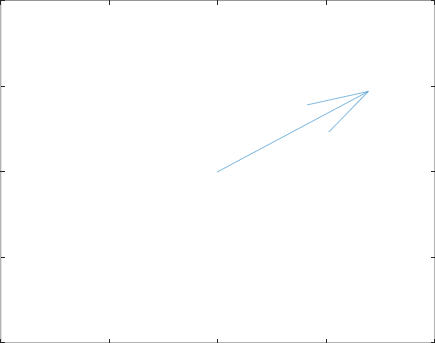## July – Animating GIFs in GNU Octave

Often it is nice to plot your results as an animation. The following code demonstrates the most effective way to write GIFs in Octave that I could find. Example code outputs an animation of a complex base-band signal symbol in noise. Code uses the quiver plot, but any other plot should do equally well. Matlab compatibility is not guaranteed.The point is to loop through all frames and save them to a file after each other with functions getframe and imwrite.

```function writeGIF()
clear all;
close all;
clear imwrite;

N = 100; %%Number of frames.

%%We use quiver plot as an example, but any other plot works equally.
signal = 5 + 5*i; %%Baseband symbol.
signal = signal + randn(1) + randn(1)*i ;   %%Add some Gaussian noise.
quiver(0,0,real(signal), imag(signal))
axis([[-10, 10],[-10, 10]])

%%Write the first frame.
frame = getframe(); %%Get the current frame.
imwrite(frame.cdata,'gifu.gif','gif','writemode','overwrite',...
'LoopCount',inf,'DelayTime',0.1, 'Compression' , 'lzw');  %%Write the frame to gifu.gif and use delay time 0.1 seconds.

for iii = 1 : N
iii
%%Write GIF.
signal = 5 + 5*i; %%Baseband symbol.
signal = signal  + rand(1) + randn(1)*i ;   %%Add some Gaussian noise.
quiver(0,0,real(signal), imag(signal))
axis([[-10, 10],[-10, 10]])

frame = getframe(); %%Get the current frame.
imwrite(frame.cdata, 'gifu.gif','gif','writemode','append','DelayTime',0.1, 'Compression' , 'lzw'); %%Write the frame to gifu.gif.
end
end
```
The 'Compression' setting is important to avoid insane file sizes and memory usage.

References: